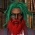Display replies flat, with oldest first Display replies flat, with newest first Display replies in threaded form Display replies in nested formDmitrii Zhelezov (Alfred Renyi Institute of Mathematics, Budapest), "An analytic approach to cardinalities of sumsets"by Egor Pifagorov - Saturday, 25 January 2020, 06:02 PM КОЛЛОКВИУМ ФАКУЛЬТЕТА МАТЕМАТИКИ И КОМПЬЮТЕРНЫХ НАУКФакультет математики и компьютерных наук, аудитория 105 (14-я линия В. О., 29)чт. 30 января 17:15Dmitrii Zhelezov (Alfred Renyi Institute of Mathematics, Budapest) An analytic approach to cardinalities of sumsets The aim of this study is to understand the nature of structures in Zd, the presence of which implies that the sumset must be large. The archetype is Freiman’s theorem that if a set A ⊂ Z d is proper d-dimensional, then|A+A|\geq (d+1)|A|-\binom{d+1}{2}. The assumption on dimension can be expressed as Sd ⊂ A for a d-dimensional simplex Sd. In general, the induced doubling of a set U is the quantity\inf\limits_{A\supset U}\frac{|A+A|}{|A|}; our main aim is to give lower estimates for it and related quantities. Applications for the sum-product problem, related to the work of [BC04], will be the subject of another paper. While our main interest is in Zd, we shall mostly formulate our results for general, typically torsion-free commutative groups. Since we work with finite sets and a finitely generated torsion-free group is isomorphic to some Zd, it is not more general, but we rarely need the coordinates. In the first part we work with sets, in the second part we study a weighted version which will be necessary for the proof of the main results. By introducing a weighted analog, we will be able to use tensorization: that is we prove a d-dimensional inequality by induction on dimension alongside a two point inequality. This is a method commonly used in analysis, for instance in the Pr´ekopa-Leindler inequality [Pr´e71] and Beckner’s inequality [Bec75]. We discuss this more below, but also invite the reader to the excellent survey paper of Gardner [Gar02]. [BC04] Jean Bourgain and Mei-Chu Chang. On the size of k-fold sum and product sets of integers. Journal of the American Mathematical Society, 17(2):473–497, 2004. [Bec75] William Beckner. Inequalities in Fourier analysis. PhD thesis, Princeton., 1975. [Gar02] Richard Gardner. The Brunn–Minkowski inequality. Bulletin of the American Mathematical Society, 39(3):355–405, 2002. [Gar02] Richard Gardner. The Brunn–Minkowski inequality. Bulletin of the American Mathematical Society, 39(3):355–405, 2002. [Pr´e71] Andr´as Pr´ekopa. Logarithmic concave measures with application to stochastic programming. Acta Scientiarum Mathematicarum, 32:301–316, 1971.